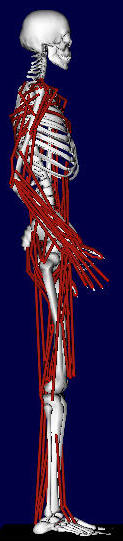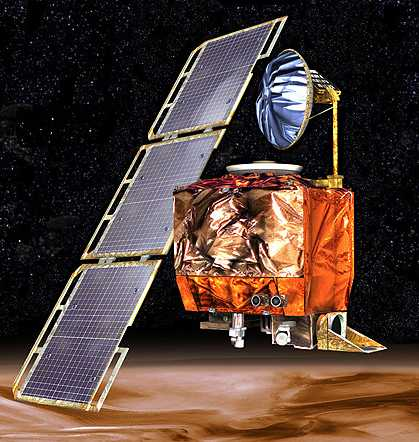Motion and Simulation Training
 Contact Motion Genesis   to schedule training or on-site seminar. Transportation and accommodations may be booked by either Motion Genesis or its client. Other courses, options, and   textbooks   are available. MotionGenesis Learning Lab (K-20). Training is usually provided by a MotionGenesis consultant. Please have estimates for the following: Event (e.g., professional training, instructor training, or academic seminar) Event type (e.g., basic/advanced motion simulation or control-system integration) Preferred schedule (several options are ideal) Desired number of software training licenses and/or textbooks Estimated budget and number of attendeesMotionGenesis: Sample 1-5 day motion and simulation training course

 Vector operations Notation: Syntactical form, constructors, RigidFrame, RigidBody. Addition/negation/subtraction: Computation, uniform basis, mixed basis. Dot and Cross: Command syntax, functions for calculating angles, distances, area, volume, ... Ordinary time-derivative: Command syntax, need for a reference frame in computation. Partial derivative: Command syntax, possible need for a reference frame in computation. Rotational kinematics Rotation matrix: Syntactical form, simple rotation matrix, successive rotations, matrix multiplication, command syntax, automated computation with syntactical forms. Angular velocity: Syntactical form, simple angular velocity, angular addition theorem, use with vector differentiation, command syntax, automated computation with syntactical forms. Angular acceleration: Syntactical form, definition, utility in formulas, command syntax, automated computation with syntactical forms. Rotational Odes: Euler angles, Euler parameters, Rodrigues parameters, Poisson parameters.Translational kinematics Position vector: Syntactical form, command syntax, automatic computation. Velocity: Syntactical form, formulas for forming velocity, computation. Acceleration: Syntactical form, formulas for forming acceleration, command syntax, automated computation with syntactical forms. Mass distribution Mass: Assigning mass of particles and bodies. Summing mass of particles, bodies, and systems. Mass center: Syntax for body's center of mass. Calculating position, velocity, and acceleration of system mass centers. Inertia properties: Assigning rigid body's via inertia dyadics, matrices, moments, and products. Calculating system inertia properties (dyadics, matrices, moments, products, and radii of gyration).Force, torque, moment, power, work, and energy Force: Syntactical form. Command syntax for adding forces to points. Command syntax for summing forces on points, particles, bodies, frames, and systems. Force models for gravity (local/universal), electrostatics, springs, dampers, etc. Torque: Syntactical form. Command syntax for adding torque to reference frames. Torque models for viscous dampers, etc. Moment: Command syntax for summing moments of forces on points, particles, bodies, frames, and systems about a designated point. Power/work: Calculating system power and work done by dissipative forces. Energy: Commands for kinetic/potential energy and energy checking functions.Statics and dynamics Translation: Command syntax for statics or dynamics using forces or Newton's equations for points, particles, bodies, frames, and systems. Rotation: Command syntax for statics or dynamics using moments or Euler's equations (angular momentum principle) for points, particles, bodies, frames, and systems. System: Command syntax for statics or dynamics of systems using generalized methods, e.g., Kane and Lagrange. Simulation and code generation (C, Fortran, MATLAB®, ...) Linear algebraic equations: Solve, Input, Output, Units, and UnitSystem. Nonlinear algebraic equations: Solve, initial guesses and convergence. Nonlinear differential equations: Integration step, error tolerances, checking functions, graphing.Constraints (part of 3+ day course) Augmented method: Augmenting constraints to equations of motion. Initial configuration and motion problems. Embedded method: Determination of independent and dependent variables. Mixed methods: Constrained systems with augmented and embedded constraints. Efficiency (part of 3+ day course) Configuration variables: Generating efficient simulation and control-systems codes. Motion variables: Choice of angular velocity variables, generalized speeds, and independent/dependent subsets. AutoZee: Automating the introduction of efficient intermediate variables.Linearization and control-system integration (part of 3+ day course) Linearization: Nominal solutions, perturbations. Efficient linearization: Efficient generation of linearized equations of motion. Stability analysis: Eigenvalues, eigenvectors, system response. Control system design: State-space feedback control techniques.